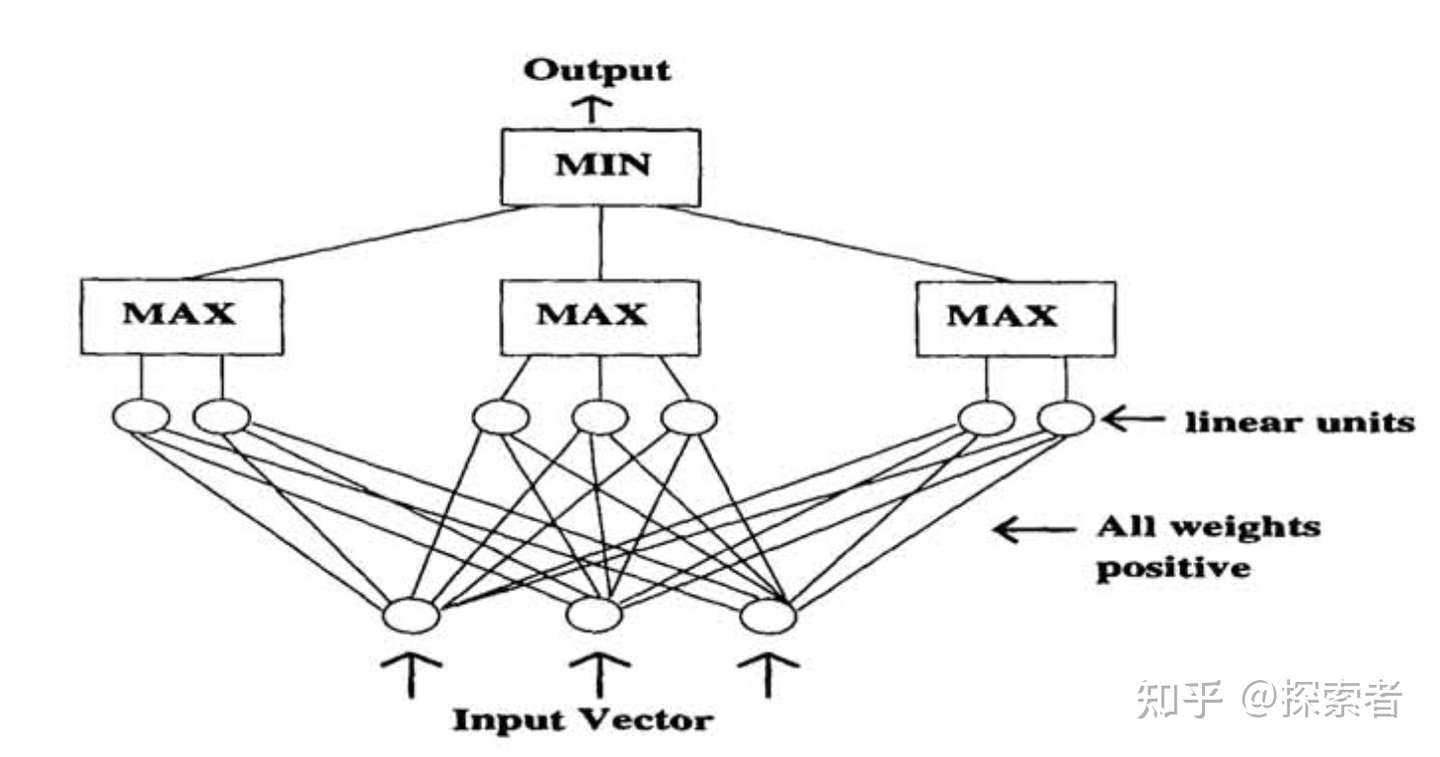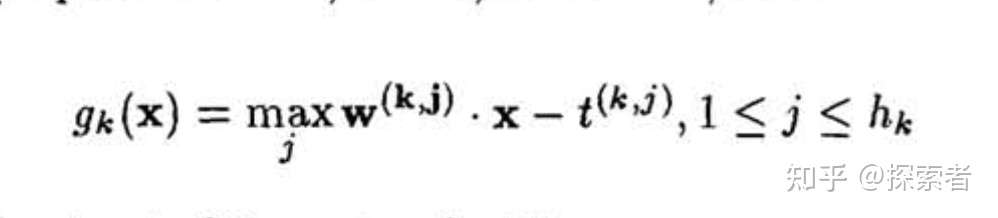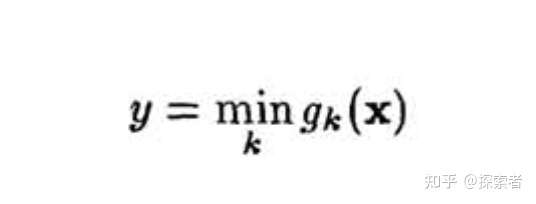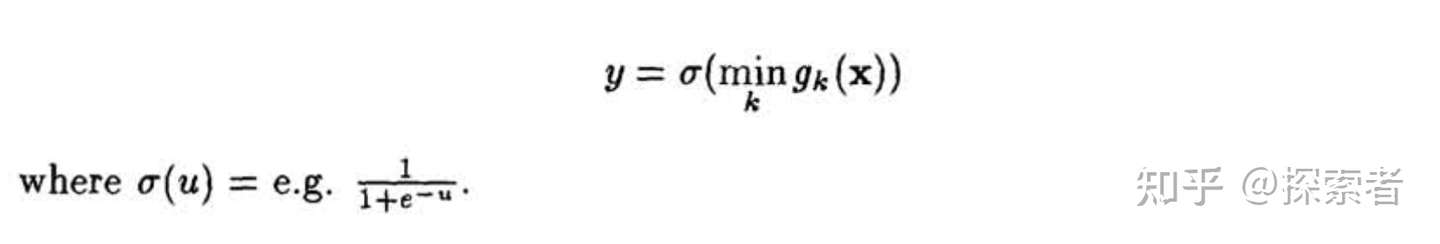# 单调神经网络《 Monotonic Networks》及代码实现

## 1.论文概述单调神经网络设计图神经网络的第一层## 2.代码实现

import torch
from torch import nn
class MNET(nn.Module):
def __init__(self):
super().__init__()
self.layer_one = nn.Linear(32, 64) #将64个神经元，分为4组,每一组有16个神经元
self.sigmoid = nn.Sigmoid()

#forward这里就是神经网络的输入了
def forward(self, input_x):
length=len(input_x)
input_x = self.layer_one(input_x)

group_one=input_x[:,:,:16] #shape:[10,1,16],一共有16组神经元
group_two=input_x[:,:,:32]
group_three=input_x[:,:,:48]
group_four=input_x[:,:,:64]

#然后取每一个group当中，输出值最大的神经元

max_one=self.find_max(group_one)
max_two=self.find_max(group_two)
max_three=self.find_max(group_three)
max_four=self.find_max(group_four)

#然后找到这4组数据的最小值.每一个数据，只选取4个数据当中的一个
max_group=torch.cat((max_one,max_two),dim=1)#横着拼
max_group=torch.cat((max_group,max_three),dim=1)
max_group=torch.cat((max_group,max_four),dim=1)

return self.find_mini(max_group)

def find_max(self,group):
#这个找最大，的确应该在每一个数据当中找最大，如果每次在喂进的所有数据当中找最大，还是不靠谱的
max_ret=torch.max(group,dim=2)
return max_ret.values

def find_mini(self,group):
mini_ret=torch.min(group,dim=1)
return mini_ret.values.unsqueeze(-1)


loss = criterion(out, var_y)
loss.backward()
optimizer.step()

for p in net.parameters():
p.data.clamp_(0, 99)


posted @ 2022-02-11 17:33  Geeksongs  阅读(916)  评论(0编辑  收藏  举报